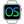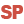HelpWLanguageWLanguage functionsStandard functionsQueue, stack, list and array management functionsArray functionsPrefix syntaxInfoPresentationExampleSee alsoAddAddLineAddReferenceAddSortedAllFalseAllTrueAnyFalseAnyTrueApplyCopyCountDeleteDeleteAllDeleteDuplicateDeleteLineDistinctFilterInfoInsertInsertLineInsertReferenceIntersectMapMaxMeanMinMoveMoveLineReduceReverseSeekSeekByProcedureShuffleSortSumSwapLineUnionUnionAllDeleteAllFunctions for managing arrays (prefix syntax)
WINDEVWEBDEVWINDEV MobileOthers<Array>.Info (Function)
In french: <Tableau>.Info
Returns the characteristics of an array: type of elements, number of dimensions, dimensions, etc.
Remark: This function can only be used on WLanguage arrays.
Example
// Total number of elements found in a one-dimensional array
MyArray is array of 5 int
...
nbDim is int
nbDim = MyArray.Info(tiTotalNumber)
// nbDim is equal to 5
// Array dimensions
MyArray is array of 5 by 2 int
nbRowDim is int
nbRowDim = MyArray.Info(tiNumberRows)
// NbRowDim is equal to 5
nbColumnDim is int
nbColumnDim = MyArray.Info(tiNumberColumns)
// NbColumnDim is equal to 2
Syntax
<Result> = <WLanguage array>.Info(<Type of information> [, <Additional parameter>])
<Result>: Integer or boolean
Requested information.
Remark: The function returns no result on a dynamic array that has not been created.
<WLanguage array>: Array
Name of Array variable to use.
<Type of information>: Integer constant
Type of information to retrieve:
 tiAssociativeWithDuplicate Boolean.True if the associative array supports the duplicatesFalse otherwise. tiDimension Integer. Number of elements found in a dimension of the array. must contain the index of the dimension whose number of elements is requested. Default value for : 1Associative array: Number of elements found in the array. tiDynamic Boolean.True if the array is a dynamic array.False otherwise.This constant is not available. tiElementDefinition Definition variable. Definition of the type of elements.This constant is not available. tiElementSize Integer. Size (in bytes) of an array element.This constant is not available. tiElementType Integer. Type of array elements. is an integer corresponding to the constants of TypeVar.This constant is not available. tiKeyType Integer. Type of keys found in the associative array. is an integer corresponding to the constants of TypeVar.Non-associative array: is equal to 0. tiNumberColumns Integer. Number of columns found in a two-dimensional array. The number of columns corresponds to the 2nd dimension of the array. If the array in not a two-dimensional array, tiNumberColumns = 0.Associative array: 0. tiNumberDimensions Integer. Number of array dimensions (between 1 and 10)Associative array: 1. tiNumberRows Integer. Number of rows found in a two-dimensional array. The number of rows corresponds to the 1st dimension of the array. If the array is not a two-dimensional array, tiNumberRows = 0.Associative array: 0. tiTotalNumber Integer. Total number of elements found in the array. tiTotalSize Integer. Total size (in bytes) of array.This constant is not available.
Additional parameter that must be specified according to the requested information.
Remarks

Equivalences

• The tiNumberRows constant is equivalent to the tiDimension constant used with <Additional parameter> = 1. The following codes are identical:
MyArray.Info(tiNumberRows)
MyArray.Info(tiDimension, 1)
• The tiNumberColumns constant is equivalent to the tiDimension constant used with <Additional parameter> = 2.
MyArray.Info(tiNumberColumns)
MyArray.Info(tiDimension, 2)

Miscellaneous

This function can be used with:
• simple arrays.
• fixed arrays.
• dynamic arrays.
• associative arrays.
Component: wd280vm.dll
Minimum version required
• Version 23